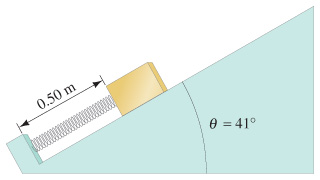# Problem: A spring (80 N/m ) has an equilibrium length of 1.00 m. The spring is compressed to a length of 0.50 m and a mass of 2.1 kg is placed at its free end on a frictionless slope which makes an angle of 41 with respect to the horizontal. The spring is then released. If the mass is not attached to the spring, how far up the slope from the compressed point will the mass move before coming to rest?If the mass is attached to the spring, how far up the slope from the compressed point will the mass move before coming to rest?Now the incline has a coefficient of kinetic friction k. If the block, attached to the spring, is observed to stop just as it reaches the springs equilibrium position, what is the coefficient of friction k?

###### FREE Expert Solution
90% (117 ratings)
###### Problem Details

A spring (80 N/m ) has an equilibrium length of 1.00 m. The spring is compressed to a length of 0.50 m and a mass of 2.1 kg is placed at its free end on a frictionless slope which makes an angle of 41 with respect to the horizontal. The spring is then released.If the mass is not attached to the spring, how far up the slope from the compressed point will the mass move before coming to rest?

If the mass is attached to the spring, how far up the slope from the compressed point will the mass move before coming to rest?

Now the incline has a coefficient of kinetic friction k. If the block, attached to the spring, is observed to stop just as it reaches the springs equilibrium position, what is the coefficient of friction k?